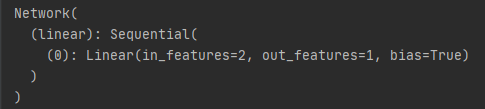# Questions about sequential. Plz help

I’m a new guy studying PyTorch and I wonder how can I get the structure with label 0 ? The following is the picture of the Network. I just want to print label 0 structure. If I use “print(net)” or “print(net[‘linear’])”, it will go wrong. I don’t know…Hi @sea_Fire,

try `net.linear`. The Sequential model is stored under the attribute `linear` in the `Network` class. Modules in a Sequential can either be selected by slicing or sometimes if there is a name given for the module by accessing the attribute via the name .

Here an example from the docs:

``````# Example of using Sequential
model = nn.Sequential(
nn.Conv2d(1,20,5),
nn.ReLU(),
nn.Conv2d(20,64,5),
nn.ReLU()
)
print(model)
print(model)
# Example of using Sequential with OrderedDict
model = nn.Sequential(OrderedDict([
('conv1', nn.Conv2d(1,20,5)),
('relu1', nn.ReLU()),
('conv2', nn.Conv2d(20,64,5)),
('relu2', nn.ReLU())
]))
print(model)
print(model, model.conv1)
``````

Thank you so much. I’m glad you replied to me.